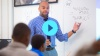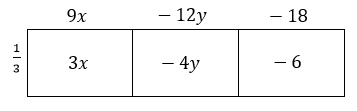# Numerical and Algebraic Expressions

Students manipulate expressions into different equivalent forms as they expand, factor, add, and subtract numerical and algebraic expressions and face authentic real-world, multi-step problems.

Math

Unit 3

## Unit Summary

In Unit 3, 7th grade students bring several prior skills together to manipulate expressions into different equivalent forms. In the preceding unit, students operated and reasoned with positive and negative rational numbers. In this unit, they use these new skills to expand, factor, add, and subtract numerical and algebraic expressions. Students pay particular attention to the structure of expressions in order to better understand what an expression means and how it can be manipulated (Standard for Mathematical Practice 7). Students also face authentic real-world, multi-step problems that require strategic use of rational numbers and estimation where appropriate.

In 6th grade, students learned how the same rules that govern arithmetic also apply to algebraic expressions. They learned to expand and factor expressions using the distributive property, and they combined terms where variables are the same. With new knowledge of the number system, students go from working with expressions like $${5(6x+3y)}$$ in 6th grade to those with rational numbers such as $${-(a+b)-\frac{3}{2}(a-b)}$$ in the 7th grade.

The next unit of the 7th grade course, Unit 4, will continue to engage students in working with expressions with rational numbers. In 8th grade, students will work with expressions and equations in both one variable and two variables, solving single linear equations and systems of linear equations. Throughout all of their future work with expressions, students’ ability to look for and make use of the structure in expressions will be as important as their ability to work with them procedurally.

Pacing: 15 instructional days (11 lessons, 3 flex days, 1 assessment day)

Fishtank Plus for Math

Unlock features to optimize your prep time, plan engaging lessons, and monitor student progress.## Assessment

The following assessments accompany Unit 3.

### Pre-Unit

Have students complete the Pre-Unit Assessment and Pre-Unit Student Self-Assessment before starting the unit. Use the Pre-Unit Assessment Analysis Guide to identify gaps in foundational understanding and map out a plan for learning acceleration throughout the unit.

### Mid-Unit

Have students complete the Mid-Unit Assessment after lesson 6.

### Post-Unit

Use the resources below to assess student understanding of the unit content and action plan for future units.

Expanded Assessment Package

Use student data to drive your planning with an expanded suite of unit assessments to help gauge students’ facility with foundational skills and concepts, as well as their progress with unit content.

## Unit Prep

### Intellectual Prep

Unit Launch

Prepare to teach this unit by immersing yourself in the standards, big ideas, and connections to prior and future content. Unit Launches include a series of short videos, targeted readings, and opportunities for action planning.#### Internalization of Standards via the Post-Unit Assessment

• Take the Post-Unit Assessment. Annotate for:
• Standards that each question aligns to
• Strategies and representations used in daily lessons
• Relationship to Essential Understandings of unit
• Lesson(s) that Assessment points to

#### Internalization of Trajectory of Unit

• Read and annotate the Unit Summary.
• Notice the progression of concepts through the unit using the Lesson Map.
• Essential Understandings
• Connection to Post-Unit Assessment questions
• Identify key opportunities to engage students in academic discourse. Read through our Teacher Tool on Academic Discourse and refer back to it throughout the unit.

#### Unit-Specific Intellectual Prep

 Model Example Rectangular array $$\frac{1}{3}(9x-12y-18)=3x-4y-6$$### Essential Understandings

• The order of operations is a guide to understanding how an expression is organized and how it can be evaluated.
• Expressions can be expanded, factored, added, and subtracted using the properties of operations and the rules of operating with rational numbers.
• Expressions can be rewritten into equivalent, often simpler forms that can be interpreted in new and different ways.

### Vocabulary

algebraic expression

combine like terms

coefficient

commutative property

constant term

distributive property

expand an expression

factor an expression

greatest common factor (gcf)

numerical expression

order of operations

To see all the vocabulary for Unit 3, view our 7th Grade Vocabulary Glossary.

## Lesson Map

Topic A: Evaluating Numerical and Algebraic Expressions

Topic B: Generating Equivalent Expressions

Topic C: Solving Multi-Step Problems using Expressions

## Common Core Standards

Key

Major Cluster

Supporting Cluster

### Core Standards

#### Expressions and Equations

• 7.EE.A.1 — Apply properties of operations as strategies to add, subtract, factor, and expand linear expressions with rational coefficients.
• 7.EE.A.2 — Understand that rewriting an expression in different forms in a problem context can shed light on the problem and how the quantities in it are related. For example, a + 0.05a = 1.05a means that "increase by 5%" is the same as "multiply by 1.05."
• 7.EE.B.3 — Solve multi-step real-life and mathematical problems posed with positive and negative rational numbers in any form (whole numbers, fractions, and decimals), using tools strategically. Apply properties of operations to calculate with numbers in any form; convert between forms as appropriate; and assess the reasonableness of answers using mental computation and estimation strategies. For example: If a woman making $25 an hour gets a 10% raise, she will make an additional 1/10 of her salary an hour, or$2.50, for a new salary of \$27.50. If you want to place a towel bar 9 3/4 inches long in the center of a door that is 27 1/2 inches wide, you will need to place the bar about 9 inches from each edge; this estimate can be used as a check on the exact computation.

#### The Number System

• 7.NS.A.3 — Solve real-world and mathematical problems involving the four operations with rational numbers. Computations with rational numbers extend the rules for manipulating fractions to complex fractions.

• 6.EE.A.2
• 6.EE.A.2.C
• 6.EE.A.3
• 6.EE.A.4

• 7.NS.A.1
• 7.NS.A.2

• 7.EE.B.4
• 8.EE.C.7
• 8.EE.C.8

• 7.G.B.4
• 7.G.B.5
• 7.G.B.6

### Standards for Mathematical Practice

• CCSS.MATH.PRACTICE.MP1 — Make sense of problems and persevere in solving them.

• CCSS.MATH.PRACTICE.MP2 — Reason abstractly and quantitatively.

• CCSS.MATH.PRACTICE.MP3 — Construct viable arguments and critique the reasoning of others.

• CCSS.MATH.PRACTICE.MP4 — Model with mathematics.

• CCSS.MATH.PRACTICE.MP5 — Use appropriate tools strategically.

• CCSS.MATH.PRACTICE.MP6 — Attend to precision.

• CCSS.MATH.PRACTICE.MP7 — Look for and make use of structure.

• CCSS.MATH.PRACTICE.MP8 — Look for and express regularity in repeated reasoning.

Unit 2

Operations with Rational Numbers

Unit 4

Equations and Inequalities

## Request a Demo

See all of the features of Fishtank in action and begin the conversation about adoption.

Yes

No

### Any other information you would like to provide about your school?